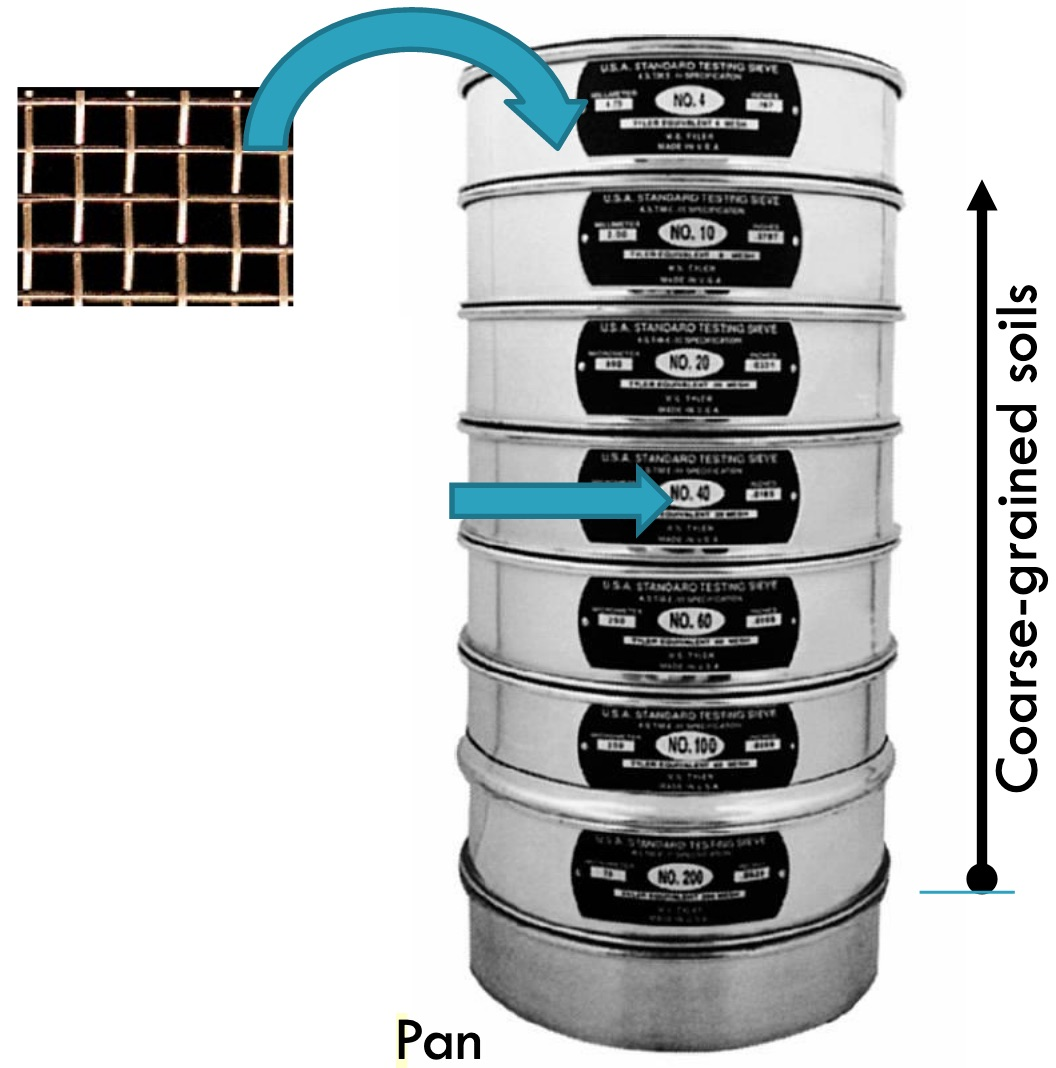Feb 26, 2016

Feb 26, 2016

Feb 26, 2016

# Sieve Analysis & Particle Size Analysis## Sieve Analysis

Sieve analysis is used to determine the grain size distribution of coarse-grained soils. For the particle size distribution of fine-grained soils, a hydrometer analysis is used. Particle size distribution is represented on a semi logarithmic plot of % finer (ordinate, arithmetic scale) versus particle size (abscissa, logarithm scale). The particle size distribution plot is used to delineate the different soil textures (percentages of gravel, sand, silt, and clay) in a soil. The effective size, D10, is the diameter of the particles of which 10% of the soil is finer. D10 is an important value in regulating flow through soils and can significantly influence the mechanical behavior of soils.

The grain size characteristics of soils that are predominantly coarse grained are evaluated by a sieve analysis. A nest of sieves is prepared by stacking test sieves one above the other with the largest opening at the top followed by sieves of successively smaller openings and a catch pan at the bottom. Opening mesh sizes of commonly used sieves are shown in table below.

Two coefficients–the uniformity coefficient and the coefficient of curvature–are used to characterize the particle size distribution. Poorly graded soils have uniformity coefficients < 4 and steep gradation curves. Well-graded soils have uniformity coefficients > 4, coefficients of curvature between 1 and 3, and flat gradation curves. Gap-graded soils have coefficients of curvature < 1 or > 3, and one or more humps on the gradation curves.

## Procedure of Particle Size Analysis:

A sample of dry soil is poured onto the top sieve, the nest is covered, and it is then shaken by hand or mechanical sieve shaker until each particle has dropped to a sieve with openings too small to pass, and the particle is retained. The cumulative weight of all material larger than each sieve size is determined and divided by the total sample weight to obtain the percent retained for that sieve size, and this value is subtracted from 100% to obtain the percent passing that sieve size. Results are displayed by plotting the percent passing (on a linear scale) against the sieve opening size (on a log scale) and connecting the plotted points with a smooth curve referred to as a grain-size distribution curve.

### Particle Size Passing the Sieves:In Sieve analysis the notation Dxx refers to the size D, in mm, for which xx percent of the sample by weight passes a sieve mesh with an opening equal to D. The D10 size, sometimes called the effective grain size, is the grain diameter for which 10% of the sample (by weight) is finer.

It is determined from the grain size distribution curve at the point where the curve crosses a horizontal line through the 10% passing value on the y axis. Other D sizes are found in a similar manner. The D50 size, called the median grain size, is the grain diameter for which half the sample (by weight) is smaller and half is larger. Two parameters are used to describe the general shape of the grain-size distribution curve.

The shape, size and distribution of particles in a soil influence:

• packing, flow of water and strength of the soil
• provide engineers with basic data to assist in selecting and using soils as construction materials.

### D10:

Effective particle size (D10) is the average particle diameter of the soil at 10 percentile; that is, 10% of the particles are smaller than this size (diameter).

### D50:

Average particle diameter (D50) is the average particle diameter of the soil.

### Cu:

Uniformity coefficient (Cu) is a numerical measure to help interpret  the distribution of particle sizes.

### Cc:

Coefficient of  curvature (Cc) is another numerical measure to help interpret  the distribution of particle sizes.

# Sieve Standards

 Sieve No. Millimeters 4 4.75 10 2.00 20 0.841 40 0.420 70 0.210 100 0.150 200 0.075
 Inches sieves Millimeters 1.5 38.1 1.0 25.4 0.75 19 0.5 12.5